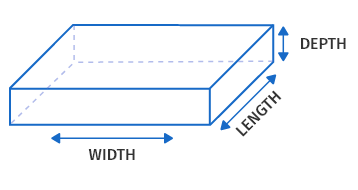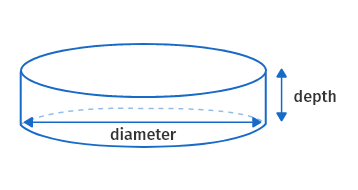# Gravel calculator

#### RESULTS

Fill the calculator form and click on Calculate button to get result here

Get Gravel calculator For Your Website

## Gravel Calculator

Gravel calculator is used to calculate the total amount of gravel you need for the construction of a house, building, or any other structure. It is a pea gravel calculator that calculates that answers the question: How much rock do I need, how much concrete do I need, or how much stone do I need? It gives you an accurate estimate in cubic yards and plans your budget considering the material, installation, and labor cost. You don't have to pay more than what you need to pay by relying on estimates from any other person or contractor.

In this post, we will explain how to use our gravel calculator, how much gravel do you need, gravel estimation for different shapes, and much more.

## How to use a gravel calculator?

The gravel calculator has two parts. You can use it to calculate the gravel quantity needed for a certain space as well as to plan the budget for your construction project. You can add as many rows as you want to find the quantity of gravel to fill up a certain space. To use gravel calculator, follow the below steps:

• Enter the number of portions in the given input box.
• Select the type of shape from the given list.
• Enter the height, length, and depth in respective input boxes.

• Enter tons per cubic yard in the given input box.
• Enter the percentage of extra material.
• Enter the cost of gravel per ton.
• Enter the labor cost per hour and other project costs in the respective input boxes.

This calculator will calculate the quantity of gravel and plan your budget in real-time. If you are wondering how much does gravel cost, you are at the right place because of the budget planning feature offered by this calculator.

## How much gravel do I need?

To fill a given area or space with gravel layers, many constructors face a lot of difficulties in estimating the required gravel quantity. Moreover, workers in the construction industry may find it difficult to come up with the exact required quantity of gravel. Our calculator lets you calculate the exact required quantity of gravel for a specific-sized area so that, you can smoothly complete your construction work without any difficulties. You can calculate the quantity of gravel needed as:

1. Identify the shape of the space for which you need gravel.
2. Use respective geometrical formulas to estimate the volume of gravel needed.
3. Determine the density of mixed gravel.
4. Multiply the density of gravel by its volume to calculate the weight.

Let's go through the process to calculate the quantity of gravel for several types of areas.

### ·Rectangular or square area

You need to multiply the gravel density by its volume to measure how much gravel you need to fill a rectangular region. The volume of a square or rectangle in cubic feet can be expressed as:

$$\text{volume}=\text{Height}\left(ft\right)\times\text{width}\left(ft\right)\times\text{length}\left(ft\right)$$

Here you can see the image of a rectangular shape:Example:

If a box has a depth of $$1 ft$$, width of $$3 ft$$, and length of $$6 ft$$, calculate the gravel required to fill it up.

Solution:

$$\text{volume}=\text{Height}\left(ft\right)\times\text{width}\left(ft\right)\times\text{length}\left(ft\right)$$

$$\text{volume}=6ft\times 3ft \times1ft = 18ft^3$$

You can multiply this volume by the density of gravel to get its weight.

### ·Round area covered with gravel

The calculation is a bit different if the shape you want to fill is round or area you want to cover with gravel like this:To calculate the volume of this shape, multiple its area to its height. Area of a round shape can be calculated using the formula:

$$A=\pi\times r^2$$, then

$$volume=A\times h$$ $$\text {or}$$ $$\pi r^2h$$

You can use our cylinder volume calculator to calculate the volume of a circular shape.

### ·Irregularly shaped area

Things can get a bit difficult if you need to calculate the quantity of gravel for an irregular shaped area. Here what you can do is:

1. Divide the irregular shape into small regular area
2. Calculate the volume for each area according to its shape.
3. Add volumes of all areas to get the quantity of gravel required to fill up the whole irregular space.

## How much is a ton of gravel?

Gravel usually costs around $$\text{\textdollar}50$$ per ton. The general price is about $$\text{\textdollar}30$$ to $$\text{\textdollar}35$$ for a cubic yard of plain pea gravel. If you need a colored variation of gravel, its cost will be up by $$\text{\textdollar}25$$  to $$\text{\textdollar}40$$. Buying gravel in bulk quantity can cut you some bucks, so consider it buying in bulk instead of small quantities.

## How much does a cubic yard of gravel weigh?

How much is a yard of gravel? How much does a yard of gravel weigh?

A cubic yard of usual gravel weighs about $$1.4$$ tons  $$2830$$ pounds. A square yard of gravel weighs about $$74kg$$ or $$57$$ pounds with a depth of $$5$$ cm or $$2$$inch.

## How many square feet does a ton of rock cover?

$$1$$ Ton of Rock Covers $$80$$ square feet if it is $$3$$" deep, $$120$$ square feet if it is $$2$$" deep, and $$240$$ square feet if the area is $$1$$" deep.

## How many yards in a ton of gravel?

A ton of gravel is about $$19$$ cubic feet or $$0.705$$ cubic yards of gravel with a normal size of stones meaning that it has been filtered and has no residual sand, clay, etc.

## Chart for Gravel and other materials weight

The below chart provides information about the weight of several landscaping materials including gravel, dry and wet topsoil, riprap, rock, dry sand, and wet sand. The weights are given in tons, kilograms, pounds, and metric tons so that you don't have to make conversion manually. You can also use our conversion calculators to make any type of conversion online.

 Material Weight per Cubic Yard Weight per Cubic Meter Pounds Tons Kilograms Metric Tons Gravel (¼” – 2″) 2,800 – 3,400 lbs 1.4 – 1.7 tons 1,660 – 2,020 kg 1.66 – 2.02 tonnes Rock (2″ – 6″) 3,000 – 3,400 lbs 1.5 – 1.7 tons 1,780 – 2,020 kg 1.78 – 2.02 tonnes Sand (wet) 3,000 – 3,400 lbs 1.5 – 1.7 tons 1,780 – 2,020 kg 1.78 – 2.02 tonnes Sand (dry) 2,600 – 3,000 lbs 1.3 – 1.5 tons 1,540 – 1,780 kg 1.54 – 1.78 tonnes Topsoil (wet) 3,000 – 3,400 lbs 1.5 – 1.7 tons 1,780 – 2,020 kg 1.78 – 2.02 tonnes Topsoil (dry) 2,000 – 2,600 lbs 1 – 1.3 tons 1,190 – 1,540 kg 1.19 – 1.54 tonnes Riprap 3,400 – 4,000 lbs 1.7 – 2 tons 2,020 – 2,370 kg 2.02 – 2.37 tonnes

Other Languages
##### Not Available for Gravel calculator
Related Calculators